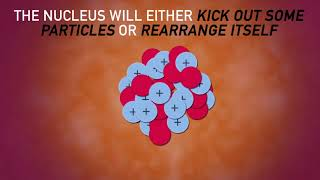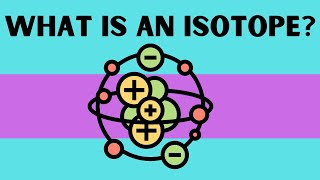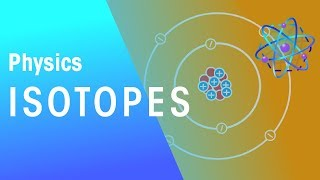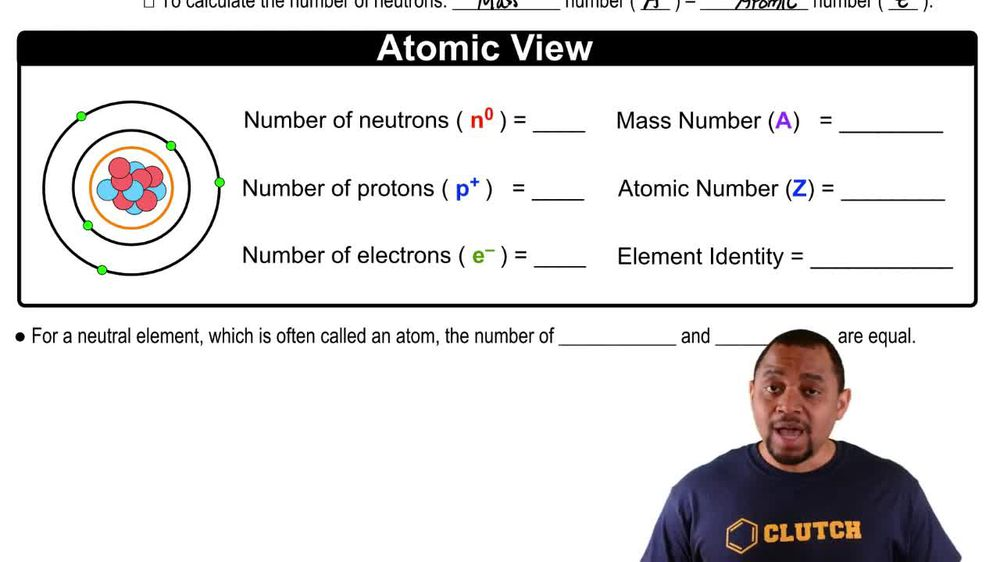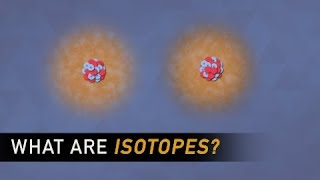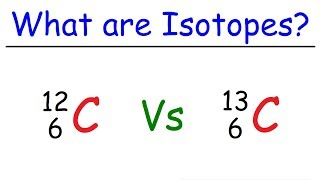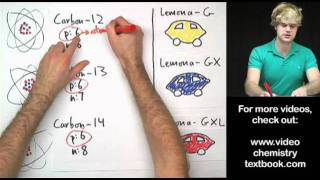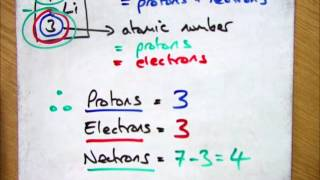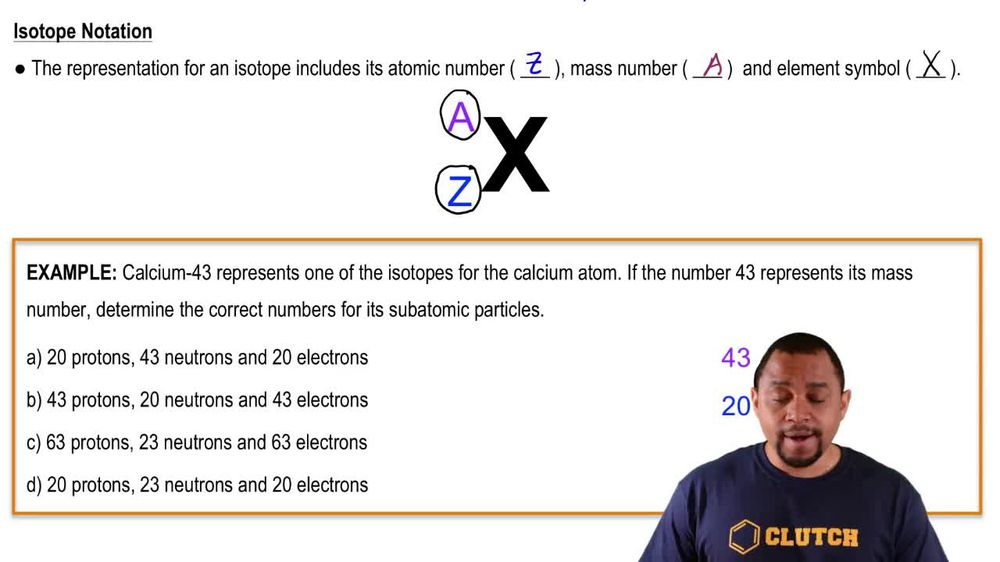Start typing, then use the up and down arrows to select an option from the list.
1. 2. Atoms & Elements2. Isotopes
Problem

# The element argon has three naturally occurring isotopes, with 18, 20, and 22 neutrons in the nucleus, respectively. (a) Write the full chemical symbols for these three isotopes.

Relevant Solution2m
Play a video:
Hey everyone, we're given a chart filled with the number of neutrons and protons for four isotopes and were asked what are their symbols? Starting with our first one. We can go ahead and use our number of protons to tell us our atomic number. In this case we have 37. So we have rubidium Now to use our neutrons. We can calculate our mass number which we can symbolize with A and this is going to be our number of protons plus the number of neutrons. In this case it'll be 37 plus 47 Which gets us to a value of 84. So our symbol here is going to be rubidium with an 84 at the top left. And at the bottom we're going to have that atomic number of 37. Next looking at our 2nd 1, our atomic number Is told to be 37 as well. So again we have rubidium. When we calculate our mass number, we get 37 Plus which gets us to that value of 85. So similar to our first question, we have rubidium but at the top left we have a mass number of 85. And at the bottom we have 37. Next looking at our third one, our atomic number Comes up to 38 And our mass number comes up to 38-plus 49 which gets us to a value of 87. Looking at our periodic table, this tells us that we have strong tm writing out our symbol, we have strontium and we have And 87 at the top left and a 38 at the bottom left. Lastly, we have our 4th one and our atomic number here Is 38 as well, which tells us we have strong team again and our mass number is going to be 38-plus 50, which gets us to a value of 88. So when we write out our symbol, we will have that 88 at the top left and at 38 at the bottom left. And these are going to be our final answers. Now, I hope it made sense and let us know if you have any questions.